# Test: Savings And Interest- 1

## 13 Questions MCQ Test Quantitative Aptitude for GMAT | Test: Savings And Interest- 1

Description
Attempt Test: Savings And Interest- 1 | 13 questions in 40 minutes | Mock test for GMAT preparation | Free important questions MCQ to study Quantitative Aptitude for GMAT for GMAT Exam | Download free PDF with solutions
QUESTION: 1

Solution:
QUESTION: 2

### An amount of 'X' dollars is invested at 'R%' simple annual interest and yields a total interest of \$100 in 2 years.In terms of X, what dollar amount invested at the same rate of interest will yield a total interest of \$400 in 4 years?

Solution:

We can create the equation:
X * R * 2 = 100
X * R = 50

R = 50/X
So to yield 400 dollars in 4 years we have:
N * 50/X * 4 = 400
N * 50/X = 100
N = 100X/50 = 2X

QUESTION: 3

### An amount X is invested in a deposit scheme which earns an annual interest rate of 8% compounded quarterly. What is the final amount after 3 years?

Solution:
QUESTION: 4

Alex invested in a Bumper scheme that pays an interest at an annual rate of 40% compounded quarterly. What is the minimum that Alex needs to invest to earn at least \$210 in interest in 6 months?

Solution:
QUESTION: 5

Alan invested \$1,000 at r% simple annual interest and an unknown amount at 2r% simple annual interest. What amount did Alan invest at 2r% simple annual interest?

1. The interest for 1 year is \$100 for 1st investment and \$200 for the 2nd investment.
2. r = 10%
Solution:
QUESTION: 6

If i investment were reduced by p percent to the new amount n, which of the following, in terms of n and p, represents the value of i ?

Solution:

Step 1: Question statement and Inferences

If i investment were reduced by p percent to the new amount n, the question is to represent the original value of i

Step 2: Finding required values

i investment went down p percent to the new amount n.

So, we can write: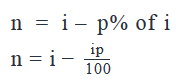Step 3: Calculating the final answer

Solve for i :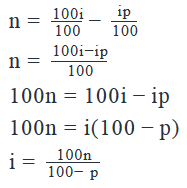QUESTION: 7

If an investment principal p decreased d percent in each of the first two years and recovered i percent in the third year, which of the following represents the value of the investment at the end of the third year?

Solution:

Step 1: Question statement and Inferences

The amount p decreased d percent each of the first two years and recovered i percent the third year. The question is to represent the value of the investment at the end of the third year.

Step 2: Finding required values

The tables below show the value of investment at the end of each year and determines the final value at the end of year 3.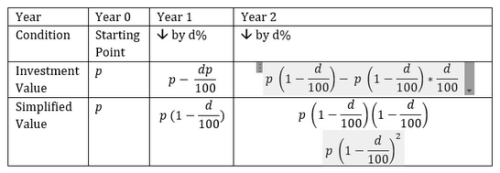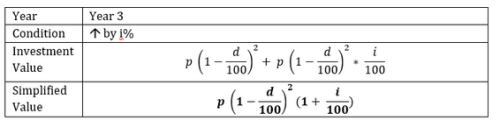QUESTION: 8

Alex bought a \$1,000 savings bond that pays interest compounded annually. If at the end of the second year, her account balance was \$1210, what is the annual rate of interest?

Solution:

Step 1: Question statement and Inferences

The bond, which started at \$1,000, is worth \$1210 after two interest cycles. What is the interest rate?

Step 2: Finding required values

Let x be the percent interest.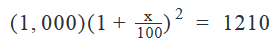Step 3: Calculating the final answer

Solve for x: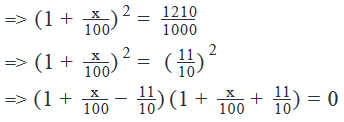The bracket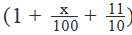yields a negative value of x. But that cannot be, because the money is growing over the two years. So, x has to be positive

So, the value of x will be obtained from: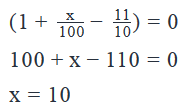Thus, the annual rate of interest is 10%

QUESTION: 9

If a total of \$10,000 is invested in two separate accounts and it earns a total of \$175 simple interest after one year, what are the annual interest rates of these two accounts?

(1) The ratio of the interest rates in two accounts is 3:4

(2) The ratio of the amounts invested two accounts is 1:1

Solution:

Step 1 & 2 – Understand and Analyze Question Statement

Knowing that \$10,000 earns \$175 from two different accounts at two different rates, set up the following equation with p as one of the principals, r1 and r­2 as the rates of interest for the two accounts.  Since the total money invested is \$10,000, second principal amount is (10,000 - p)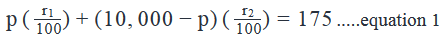With one equation and three unknowns (p, r1, and r2), we can’t solve the equation.

Step 3 – Analyze Statement 1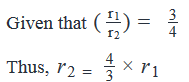Place these in equation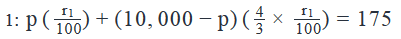With one equation and two unknowns (p and r1

), we can’t solve the equation. Statement 1 is not sufficient.

Step 4 – Analyze Statement 2

Statement 2 tells us that \$10,000 is evenly split. Place these in equation 1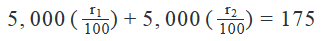With one equation and two unknowns, we still can’t solve the equation. Statement 2 is not sufficient.

Step 5 – Analyze Statements 1 and 2 Together

Taken together, we have a single equation with one unknown, and hence we can solve for  :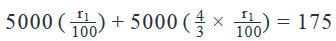Note that we do not need to do the calculation to answer this question.  But if you perform the calculation, the answer is as follows:

r1=1.5%

; r2=2.0%

QUESTION: 10

\$2000 is invested in a new savings account at r percent interest rate compounded annually. If the amount in the account after 3 years is \$3456, what is the total interest (in dollars) earned after 2 years of starting the account?

Solution:

Step 1: Question statement and Inferences

We are given that a sum of \$2000 is invested in a new savings account. This savings account offers an interest rate of r percent, which is compounded annually.

The balance in the account at the end of three years is \$3456.

We have to find the total interest earned after 2 years.

We know that the formula for compound interest is: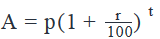Here,

A = Final amount at the end of time t = \$3456

p = Initial Amount = \$2000

r = rate of interest

t = number of compounding periods ‘n’ in the total duration = 3 years

Step 2: Finding required values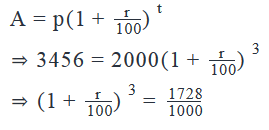Upon breaking down 1728 into its factors, the above equation becomes: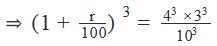Taking cube root on both sides: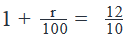Upon simplifying this equation further, we get: r = 20%

Now, we have to find the interest earned at the end of 2 years. To find this, we first need to find the amount that the initial deposit will have grown to after 2 years: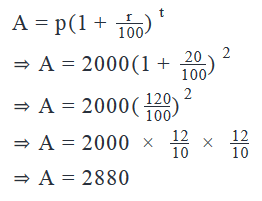Step 3: Calculating the final answer

Interest earned after 2 years = (Amount after 2 years) – (Initial amount)

= 2880 – 2000

= 880

Thus, the interest earned after 2 years will be \$880

QUESTION: 11

What will be the value of \$1000 at the end of 3 years?

(1)   The return for the investment is 10% p.a., compounded annually.

(2)   The amount at the end of the first year is \$1100.

Solution:

In order to find the value of \$1000 at the end of 3 years, we need the rates of return for each of the three years.

Let the rate of return for the first year be r1 %

So, the amount at the end of the first year, A1 = 1000 + r1 % of 1000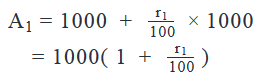Let the rate of return for the second year be r2 %

So, the amount at the end of the second year, A2 = A1 + r2 % of A1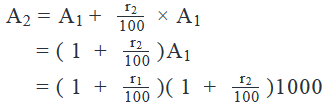Let the rate of return for the third year be r3 %

We can similarly prove that the amount at the end of the third year,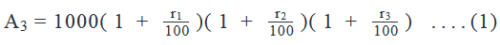Thus, in order to find the amount at the end of the third year, we need to know the values of r1, r2 and r3

Step 3: Analyze statement 1

Statement 1 says that:

The annual rate of return for the investment is 10%

This statement implies that the rate of return for each of the three years is 10%

Putting r1 = r2 = r3 = 10 in Equation 1: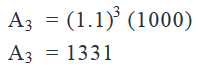Thus, using Statement 1, we are able to find a unique value for

So, Statement 1 is sufficient

Step 4: Analyze Statement 2

Statement 2 states that

The amount at the end of the first year is \$1100

From this statement, we do not know if the interest offered was CI or SI. Also, if it was CI, we do not know the compounding frequency.

Thus, Statement 2 is not sufficient.

Step 5: Analyze Both Statements Together (if needed)

This step is not needed as we do get a unique answer in Step 3

So, the correct answer is Option A.

QUESTION: 12

What is the approximate amount that should be invested today at an annual rate of 10% compounded semi-annually so that an amount of \$1000 is received at the end of the first year?

Solution:

Step 1: Question statement and Inferences

This question tests your proficiency in solving problems related to compound interest.  Please note the formula used for compound interest problems: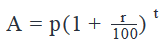Here A = \$1000;  r = 10% compounded semi-annually;  t = compounding time intervals

Step 2: Finding required values

r is given as annual rate of interest.  Since we know that the interest is compounded semi-annually.  So the rate for calculation = r/2 = 10/2 = 5%.  Also, the time intervals will be 2 since the compounding will be done 2 times till the end of first year. So t = 2.

Step 3: Calculating the final answer

Substituting these values we get: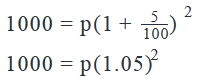Calculating and approximating (1.05)2, we get:

1000 = p × 1.1

Thus, p = 1000/1.1 = 909.09

Thus the closest answer is \$910.

QUESTION: 13

If p were invested at i annual interest rate compounded annually for t years, where t > 3, and at the start of the second last year, the annual interest rate was doubled and then maintained at that level till the end, which of the following represents the amount received at the end of t years?

Solution:

Step 1: Question statement and Inferences

Based on the compound interest equation: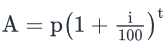, you are asked to represent the amount when the rate of interest changes. The interest rate is i for the first (t-2) years, then 2i for the last two years.

Step 2: Finding required values

Start with the amount calculation for the first set of years, before the interest changes. If t is the total number of years, this set of years is t – 2.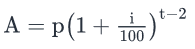Next, calculate the amount for the final two years, when the interest becomes 2i. In this case, t equals 2. Note that the principal for this time interval is the Amount calculated above.  Thus, the amount at the end of t years is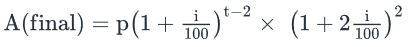Use Code STAYHOME200 and get INR 200 additional OFF Use Coupon Code